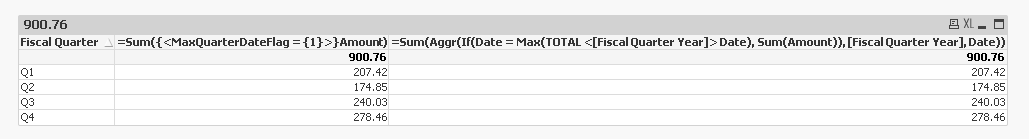# QlikView App Dev

Discussion Board for collaboration related to QlikView App Development.

Announcements
Welcome to Qlik Community! Check out our new navigation! FIND OUT MORE
cancel
Showing results for
Did you mean:Partner - Creator

## Amount at Maximum date of quarter

Hi,

I have data at every date for the current fiscal year. And I wants the data as on maximum date of respective quarter.

I have attached the raw data format and desired output.

Kindly suggest expression.

1 Solution

Accepted SolutionsMVP

Two options

1) Create flag in the script like this

Table:

[Fiscal Quarter],

Amount,

[Fiscal Quarter] & Year(YearStart(Date, 0, 4)) as [Fiscal Quarter Year],

Year(YearStart(Date, 0, 4)) as [Fiscal Year]

FROM

(ooxml, embedded labels, table is Data);

Left Join (Table)

LOAD [Fiscal Quarter Year],

Max(Date) as Date,

1 as MaxQuarterDateFlag

Resident Table

Group By [Fiscal Quarter Year];

and then use this expression

=Sum({<MaxQuarterDateFlag = {1}>}Amount)

2) Use this expression without adding flag in the script

=Sum(Aggr(If(Date = Max(TOTAL <[Fiscal Quarter Year]> Date), Sum(Amount)), [Fiscal Quarter Year], Date))

or if you only have single year data, then this

=Sum(Aggr(If(Date = Max(TOTAL <[Fiscal Quarter]> Date), Sum(Amount)), [Fiscal Quarter], Date))4 RepliesSpecialist

I hope this helps,

Cheers,

LuisMVP

Two options

1) Create flag in the script like this

Table:

[Fiscal Quarter],

Amount,

[Fiscal Quarter] & Year(YearStart(Date, 0, 4)) as [Fiscal Quarter Year],

Year(YearStart(Date, 0, 4)) as [Fiscal Year]

FROM

(ooxml, embedded labels, table is Data);

Left Join (Table)

LOAD [Fiscal Quarter Year],

Max(Date) as Date,

1 as MaxQuarterDateFlag

Resident Table

Group By [Fiscal Quarter Year];

and then use this expression

=Sum({<MaxQuarterDateFlag = {1}>}Amount)

2) Use this expression without adding flag in the script

=Sum(Aggr(If(Date = Max(TOTAL <[Fiscal Quarter Year]> Date), Sum(Amount)), [Fiscal Quarter Year], Date))

or if you only have single year data, then this

=Sum(Aggr(If(Date = Max(TOTAL <[Fiscal Quarter]> Date), Sum(Amount)), [Fiscal Quarter], Date))Partner - Creator
Author

Thanks for your help..Partner - Contributor III

Hi!  This got me exactly what I needed!  Thank you for posting.  However one question...

=Sum(Aggr(If(Date = Max(TOTAL <[Fiscal Quarter Year]> Date), Sum(Amount)), [Fiscal Quarter Year], Date))

I'm using the above formula but I would only like to see the past 12 months (so the past 4 quarters).  How would I adjust to show that?